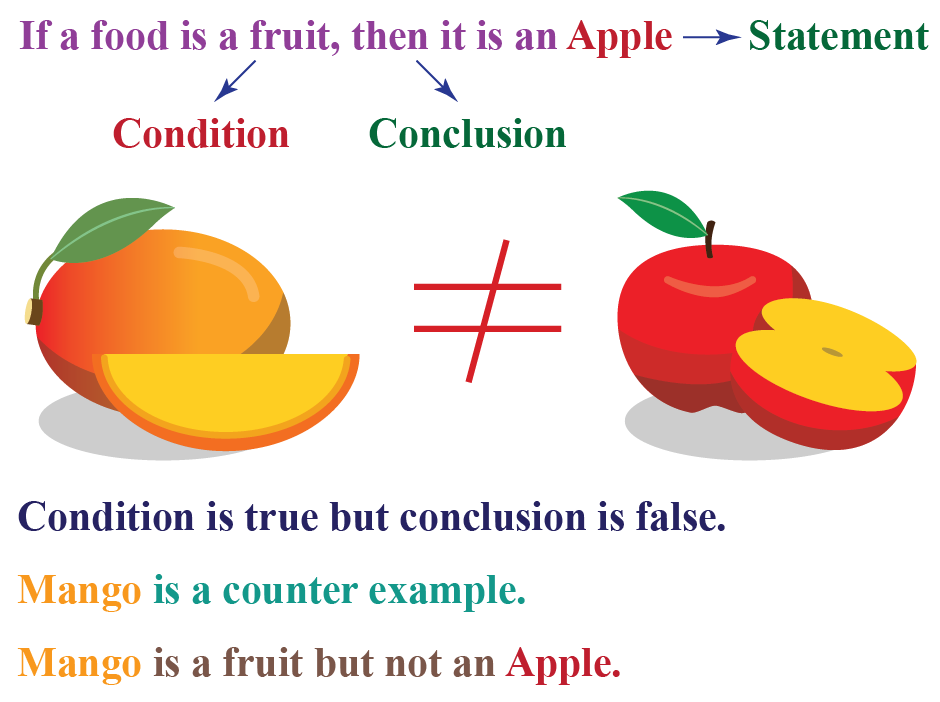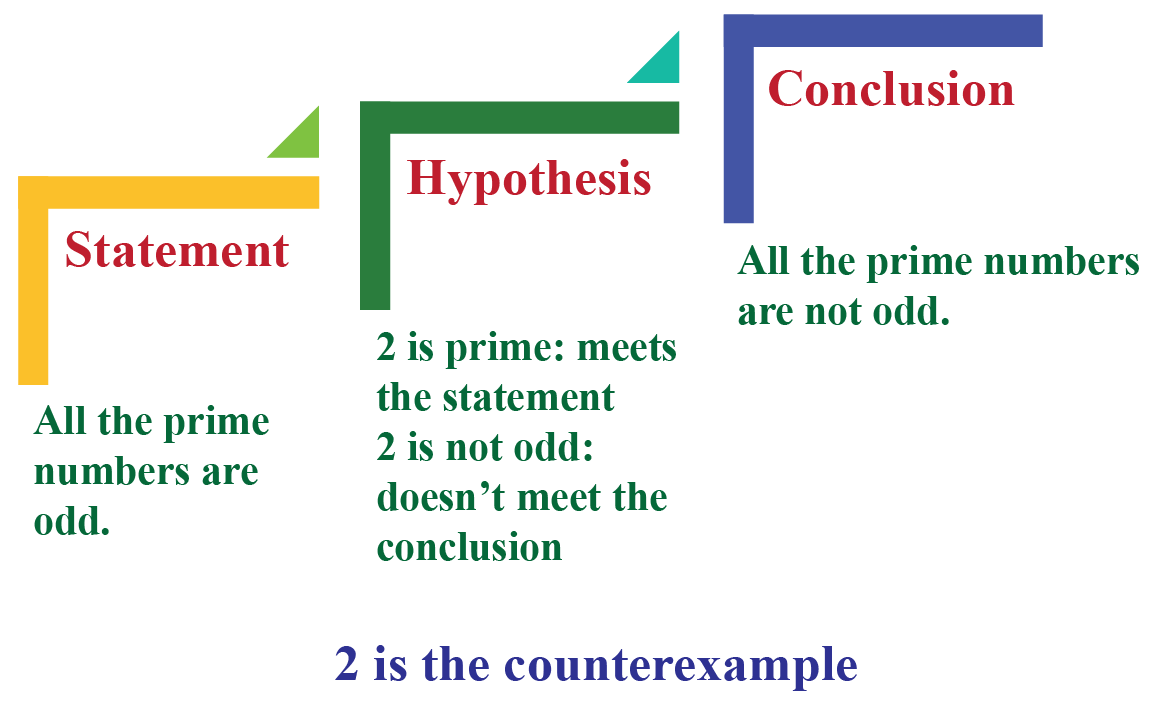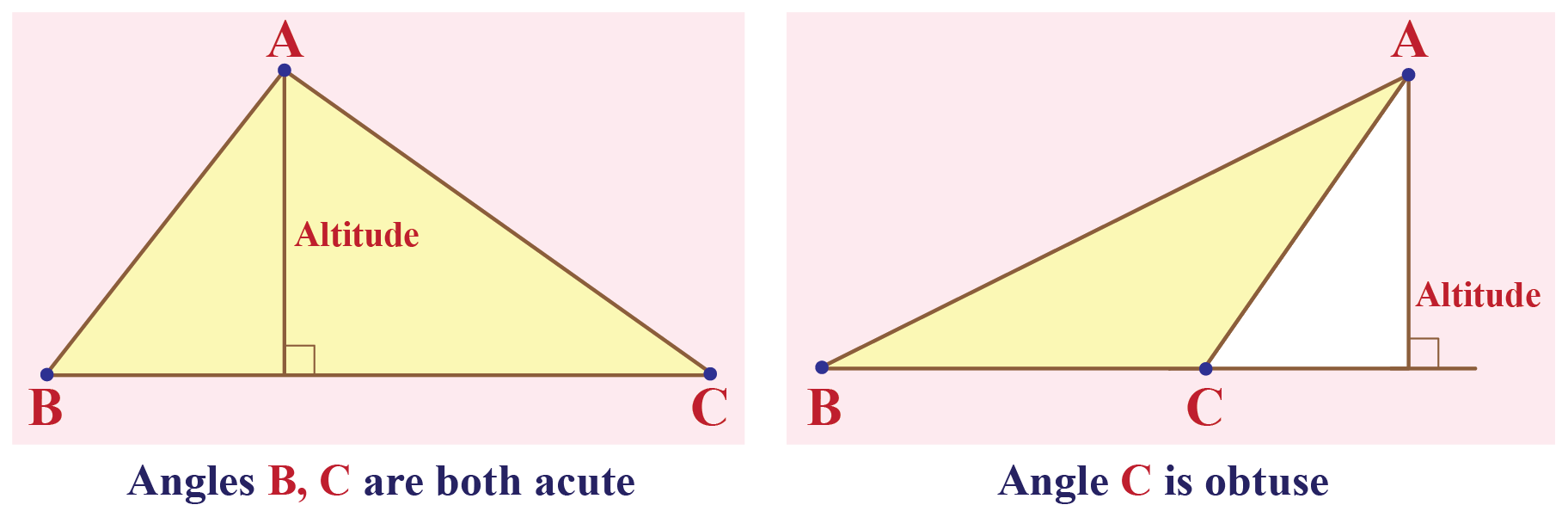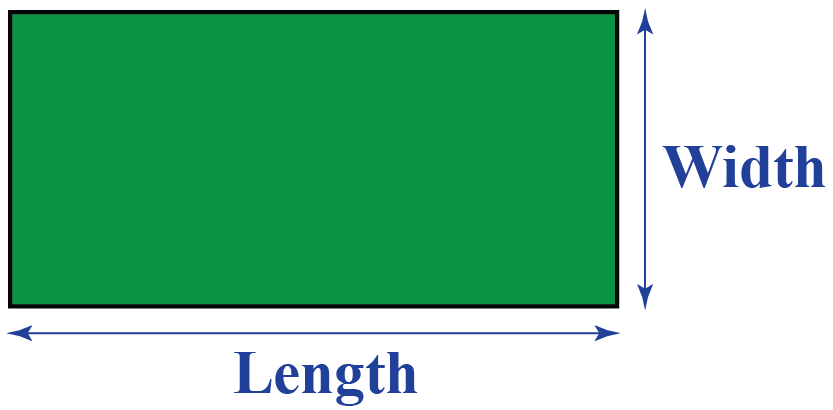# Counterexample

Counterexample

A counterexample is used to check the validity of an argument. Consider the following statement: If a food is a fruit, then it is an apple. Now, consider this statement: Mango is a food. It is a fruit, but it is not an apple. Therefore, the mango is the counterexample, thereby making the first statement invalid.

Counterexamples are used in math to contradict a statement. Counterexamples are used to prove the limitations of possible theorems.## Lesson Plan

 1 What is a Counterexample? 2 Important Notes on Counterexample 3 Solved Examples on Counterexample 4 Challenging Questions on Counterexample 5 Interactive Questions on Counterexample

## What is a Counterexample?

### Counterexample Definition

A counterexample is an example that meets the mathematical statement's condition but does not lead to the statement's conclusion.

### Counterexample Math

Instead of showing that the statement is true, we show that the statement is false using the counterexample.

Teacher: Hey Benny, do you know about prime numbers?

Benny: Prime numbers are those numbers that are divisible by 1 and itself only.

Teacher: You are right! They cannot be factored further. What else can you say about the prime numbers?

Benny: All the prime numbers are odd.

Ron: But, the prime number 2 is even.

Teacher: 2 is a prime number. It meets the statement but does not meet the conclusion that it is odd. Thus 2 is the counterexample.Thus the counterexample helps us in disproving the mathematical conjectures.

## How can we identify Counterexamples?

Counterexamples are used to prove that a statement is invalid.

Identify the hypothesis and the conclusion in the given statement.

The counterexample must be true for the hypothesis but false for the conclusion.

For example, let's identify the number 14 as a counterexample for which of the following conditional statements.

Statement Hypothesis Conc. True/ False
a) If a number is even, then it ends with 2, 4, 6, 8, or 0. The Number is even The number ends with 2, 4, 6, 8, or 0 14 is even and ends with 4.
b) If a number is divisible by 2, it is also divisible by 4. The number is divisible by 2 The number is also divisible by 4 14 is divisible by 2,
but not divisible by 4.
c) If a number is divisible by 2, then it is even. The number is divisible by 2 The number is even. 14 is divisible by 2,
and is even.
d) If a number is odd, then it's not divisible by 2. The Number is odd The number is not divisible by 2 14 is not odd
and is divisible by 2.

A counterexample is true for the hypothesis but false for the conclusion. Thus option 2 is the correct answer.

$$\therefore$$, 14 is the counterexample for the 2nd statement that states  "If a number is divisible by 2, it is also divisible by 4."

### Counterexample Calculator

Move any of the points on the left triangle every time you start with. Move any points on the right triangle: angle A' will always be congruent to angle A, and angle B' will always be congruent to B.Try connecting the dots on the right triangle in a way that makes a triangle that is NOT similar to ABC?  If you create a triangle that is similar to ABC, you can see the ratios of the sides, in this counterexample calculator. Can you make them be not quite equal?

## How do You Make a Counterexample?

'If' part must be true and 'then' part must be false.

If a number is a perfect square, then it is odd.

Let us try to set up a counterexample for this.

• 'If' part is a number that is a perfect square.

Think of 4 which is a perfect square.

This has become true.

• 'Then' part is that the number should be odd. 4 is even.

This has become false.

Thus we have set up a counterexample for the statement.

### Counterexample Geometry

Counterexamples are used in geometry to prove the conditional statements false.

1) Conjecture: "All quadrilaterals of equal length are squares".

The counterexample is a rhombus.

A rhombus has all sides of equal length, however, the angles are not straight and thus it is not a square.

2) Conjecture: "The height of a triangle lies inside the triangle".

An altitude of a triangle is perpendicular from a vertex to the opposite side.

It can also be at the outside of the triangle if the angle $$\geq 90 ^\circ$$.## What is a Counterexample to The Conditional Statement?

A counterexample is an example in which the condition is true, but the conclusion is false.

If you can find a counterexample to a conditional statement, then that conditional statement is false.

### Counterexample in Algebra

Statement: Example: If $$a^2 > b^2$$, then a > b The hypothesis is formed. $$5^2 > 2^2$$  25 > 4 and 5 > 2 The condition is true and the conclusion is true. $$(-5)^2 > 2^2$$ 25 > 4 but -5 $$\ngtr$$ 2 The condition is true but the conclusion is false. $$\therefore$$ If $$a^2 > b^2$$, then $$a>b$$ is not true. Counter example makes the statement invalid

## Solved Examples

 Example 1

Jim says “If n is an integer and $$n^2$$ is divisible by 4, then n is divisible by 4.”

Prove that he is wrong.

Solution

To give a counterexample, we need to find the square of an integer such that it is divisible by 4

Let's try with 6!

$$6^2$$ is divisible by 4 but 6 is not divisible by 4

Thus n = 6 is a counterexample to Jim's statement.

 $$\therefore$$ n = 6 is the counterexample
 Example 2

Raymond tells "If a perimeter of a rectangle is 14, then its area is 10."

Which of the following could be the counterexamples? Justify your decision.

a) a rectangle with sides measuring 2 and 5

b) a rectangle with sides measuring 10 and 1

c) a rectangle with sides measuring 1 and 5

d) a rectangle with sides measuring 4 and 3Solution

Find which disproves the statement

a) Rectangle with sides 2 and 5: Perimeter = 14 and area = 10

Both 'if' and 'then' are true. So it is not a counterexample.

b) Rectangle with sides 10 and 1: Perimeter = 22 and area = 10

Both 'if' is false and 'then' is true. So it is not a counterexample.

c) Rectangle with sides 1 and 5: Perimeter = 12 and area = 5

Both 'if' and 'then' are false. So it is not a counterexample.

c) Rectangle with sides 4 and 3: Perimeter = 14 and area = 12

Both 'if' is true and 'then' is false. So it is a counterexample.

 $$\therefore$$ Rectangle with sides 4 and 3 is the counterexample for the given statement.
 Example 3

Colin examined the set of numbers in the box are multiples of 3. She claimed that they are divisible by 9. Do you agree or disagree? Justify your answer.

 16 27 24

Solution

If a number is a multiple of 3, then it is divisible by 9 is the statement given by Colin.

To disprove let us find which is the counterexample among these.

a) 16 is not a multiple of 3. Thus the condition is false.

16 is not divisible by 9. Thus the conclusion is false.

So 16 is not a counterexample.

b) 27 is a multiple of 3.Thus the condition is true.

It is divisible by 9.Thus the conclusion is true.

So 27 is not a counterexample.

c) 24 is a multiple of 3. Thus the condition is true.

16 is not divisible by 9. Thus the conclusion is false.

So 24 is a counterexample.

 $$\therefore$$, 24 is the counterexample for the given statement.Challenging Questions
• Choose a number.  Double it.  Add 6.  Double again.  Subtract 4.  Divide by 4.  Subtract 2.
• a) Make a conjecture about the relation between the number you picked and the final result.
• b) Can you find a counterexample to your conjecture?  What does this imply?

## Interactive Questions

Here are a few activities for you to practice. Select/Type your answer and click the "Check Answer" button to see the result.

## Let's Summarize

The mini-lesson targeted the fascinating concept of counterexample. The math journey around counterexample starts with what a student already knows, and goes on to creatively crafting a fresh concept in the young minds. Done in a way that not only it is relatable and easy to grasp, but also will stay with them forever. Here lies the magic with Cuemath.

At Cuemath, our team of math experts is dedicated to making learning fun for our favorite readers, the students!

Through an interactive and engaging learning-teaching-learning approach, the teachers explore all angles of a topic.

Be it worksheets, online classes, doubt sessions, or any other form of relation, it’s the logical thinking and smart learning approach that we, at Cuemath, believe in.

## 1. What is the purpose of The Counterexample?

Disproving by counterexample is the technique in maths where a statement is shown to be invalid by finding a single example whereby it is not satisfied.

## 2. Does a  Counterexample always disprove a conjecture?

Yes. When examples prove the proof or therom or a conjecture, counterexamples disprove a conjecture.

## 3. How Counterexample helps in solving problems?

Using the counterexamples, we can produce meaningful statements and theorems.

More Important Topics
Numbers
Algebra
Geometry
Measurement
Money
Data
Trigonometry
Calculus
More Important Topics
Numbers
Algebra
Geometry
Measurement
Money
Data
Trigonometry
Calculus
Learn from the best math teachers and top your exams

• Live one on one classroom and doubt clearing
• Practice worksheets in and after class for conceptual clarity
• Personalized curriculum to keep up with school